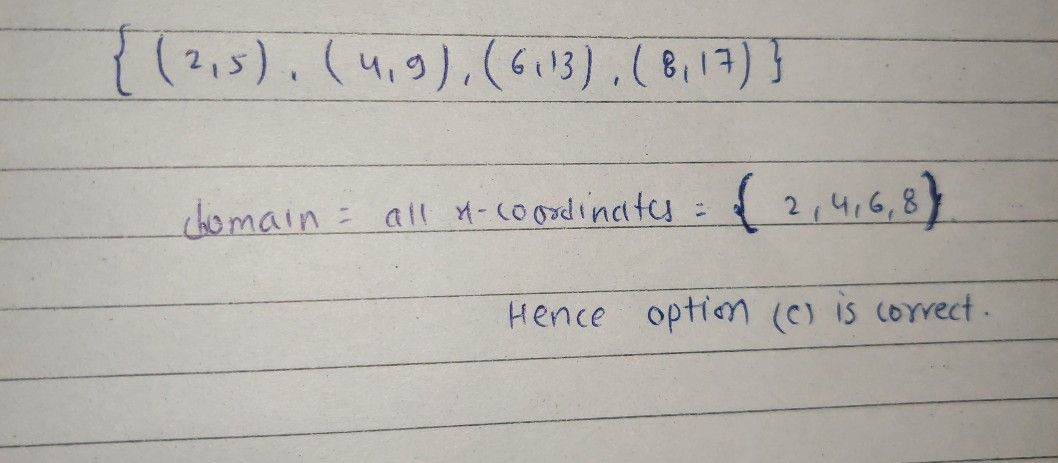Symbol
ProblemA function is given by the set of ordered pairs $\left(\left(2,5\right),\left(4,9\right),\left(6,13\right),\left(8,17\right)\right)$ What is the domain of the set of ordered pairs? $\left(5.9.13.17$ $\left(5.9,13.17\right)$ $\left(2.4.6,8\right)$ $\left(2.4.6.8\right)$
10th-13th grade
Algebra
SolutionQanda teacher - Aryanhi dear feel free to ask queries please evaluate the answer if satisfied
Please give 3000 coins as thank you gift this will not reduce your coins
happy Learning ?❣️?